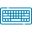# Euler's Method Calculator## Table of Content

Feedback

The Euler’s Method Calculator is utilized to approximate solutions to differential equations by implementing the Euler's method, which is a first order numerical method to solve first degree differential equation with a given initial value.

## What is the Euler Method?

“The first order numeric technique that is utilized to approximate the solutions of ordinary differential equations (ODE) of a given value is known as Euler's method”.

This method is taken name by the name of Swiss mathematician Euler. It involves estimating the values of unknown functions at discrete points and breaking down differential equations into smaller steps.

## Euler’s Method Formula:

Euler’s method formula is a simple iterative approach that determines the first order ordinary differential equations. The formula essentially updates the values of x and y at each step, moving along the curve defined by the differential equation in discrete increments.

Use the following formulas iteratively to calculate the next approximations of x and y:

$$x_{n+1} = x_n + h$$

$$y_{n+1} = y_n + h . f(x_n,y_n)$$

Euler's Method proceeds as follows:

1. Choose an initial point ( x₀, y₀ ), in which x₀ is the initial value of x, and y₀ is the initial value of y.
2. Choose small step size h to enhance x by.Euler’s method calculator starts by evaluating the slope of the tangent line to the solution curve at the initial point and after that uses this slope to determine the solution at the next point.

## Practical Example:

Let's say we have a simple function (x^2+4y)^(1/2)  that needs to approximate the value of y at x = 2, given the initial condition y(0) = 1 with a step size (h) of 0.5

### Solution:

#### To Find:

y(2) for $$y' = \sqrt{x^{2} + 4 y}$$ when h = 0.5 and y(0) = 1

Now, we'll iteratively apply Euler's Method:

$$y_{n+1} = y_n + h . f(x_n,y_n)$$

Now, we have

$$h=0.5,x_0 = 0, y_0 = 1, f(x,y)=\sqrt{x^{2} + 4 y}$$

#### Step 1:

$$x_1 = x_0 + h = 0 + 0.5 = 0.5$$

$$y(x_1) = y(0.5) = y_1 = y_0 + h. f(x_0,y_0) = 1 + 0.5 \times f(0,1)$$

$$= 1 + 0.5 \times 2.0 = 2.0$$

#### Step 2:

$$x_2 = x_1 + h = 0.5+0.5=1$$

$$y(x_2) = y(1) = y_2= y_1 + h. f(x_1,y_1) = 2.0 + 0.5 \times f(0.5,2.0)$$

$$= 2.0 + 0.5 \times 2.8722813232690143 = 3.436140661634507$$

#### Step 3:

$$x_3 = x_2 + h = 1+0.5=1.5$$

$$y(x_3) = y(1.5) = y_3= y_2 + h. f(x_2,y_2) = 3.436140661634507 + 0.5 \times f(1,3.436140661634507)$$

$$= 3.436140661634507 + 0.5 \times 3.8398649255589743 = 5.356073124413994$$

#### Step 4:

$$x_4 = x_3 + h = 1.5 + 0.5 = 2$$

$$y(x_4) = y(2) = y_4= y_3 + h. f(x_3,y_3) = 5.356073124413994 + 0.5 \times f(1.5,5.356073124413994)$$

$$= 5.356073124413994 + 0.5 \times 4.865623546643942 = 7.788884897735965$$

$$y(2) = = 7.788884897735965$$

#### Numeric Solution In Table:

The below table helps to make your Euler's method calculator more engaging and informative.

Step x0 y0 slope yn
1 0 1 2.0 2.0
2 0.5 2.0 2.8722813232690143 3.436140661634507
3 1 3.436140661634507 3.8398649255589743 5.356073124413994
4 1.5 5.356073124413994 4.865623546643942 7.788884897735965

This demonstrates how Euler's formula calculator efficiently approximates solutions to differential equations, making complex problems more manageable.

## How Euler’s Method Calculator Function?

Euler method calculator are typically used to solve simple differential equations and also to generate data for plotting the solution to differential equations. Solve differential equations with ease and precision by putting the below values.

#### Start by Filling in:

• Insert the function and mention the step size (h) or the number of steps used in the calculation.
• Put the value for y and the initial conditions

#### Results Summary:

Our online Euler's calculator with step size gives you the final results after inserting the above values in it.

• Approximated Solution: Display the final value of the unknown function or variable (y) at the endpoint (x).
• Step Size (h): Show the step size (h) and explain that smaller step sizes can lead to more accurate results but may require more iterations.
• Initial Conditions: Present the initial conditions provided by the user, including the initial values of x (x₀) and y (y₀).
• Number Steps in Table: This table can enable understanding the number of iterations or steps taken to reach the endpoint can enable understand

## List of Resources:

Wikipedia: Euler method, Geometrical description, Derivation, Local truncation error, Global truncation error.

LibreTexts: Euler's Method, Examples Illustrating The Error in Euler’s Method, Truncation Error, Semilinear Equations and Variation of Parameters.### Alan Walker

Studies mathematics sciences, and Technology. Tech geek and a content writer. Wikipedia addict who wants to know everything. Loves traveling, nature, reading. Math and Technology have done their part, and now it's the time for us to get benefits.